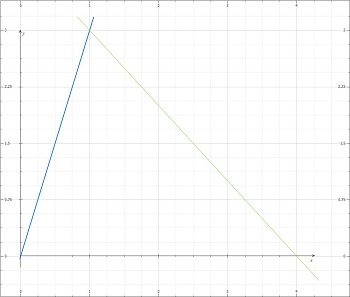# Let C be the boundary of the triangle with vertices at (0, 0), (1, 3), (4, 0) traversed in the...

## Question:

Let C be the boundary of the triangle with vertices at (0, 0), (1, 3), (4, 0) traversed in the clockwise direction.

(a) Sketch C.

(b) Evaluate the line integral.

{eq}\int_{C}[\log(1 + y) + xy] dx + \frac{x}{1 + y} dy. {/eq}

## Line Integrals, Green's Theorem:

A line integral around a curve may be computed utilizing Green's Theorem, if the hypothesis of the theorem is satisfied:

{eq}\text{Green's Theorem:} {/eq}

Let C be a piecewise smooth simple closed curve that bounds the region R in the plane. Suppose that the functions P(x,y) and Q(x,y) are continuous and have continuous first-order partial derivatives in R. Then,

{eq}\displaystyle \oint_C P \; dx + Q \; dy = \iint_R \left(\frac{\delta Q}{\delta x} - \frac{\delta P}{\delta y}\right) \; dA {/eq}.

Line integrals find several applications a few which are, computing the mass of a wire or the center of mass, moment of inertia of a plane region, as well as the area of the region.

Become a Study.com member to unlock this answer! Create your account

C is the boundary of the triangle with vertices at (0, 0), (1, 3), (4, 0) traversed in the clockwise direction.

(a)Observe the graph of C.(b)...Line Integrals: How to Integrate Functions Over Paths

from

Chapter 15 / Lesson 2
1.6K

Many real-world functions are three dimensional, as we live in a 3D world. In this article, you will learn how to integrate 3D functions over general paths through space. This is a basic skill needed for real science and engineering applications.